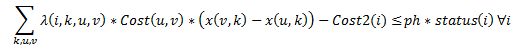# Complex Constraint

Dear Friends,
I want to express the following constraint:
I’ve tried to write the following constraint (benders optimality cut)(x is a binary variable)expression temp(size_i,size_k,size_u,size_v);
for I=1: size_i
for k=1: size_k
for u=1: size_u
for v=1: size_v
if(v_net_topology(u,v)==1)
temp(I,k,u,v)=lambda{I}(k,u,v)cost(u,v)(x(v,k)-x(u,k));
end
end
end
end
end

``````for I=1:size(Object2,2
sum(sum(sum(temp(I,:,:,:))))-cost2(I)<=ph*STATUS{i};
end
``````

It works without any error, but in the next iterations, it returns the same x. I think I didn’t use the expression correctly. Am I right?

Dear Michael @mcg
I’m unable to edit my pot. It returns 403 forbidden!